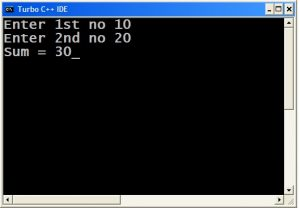# Basic C Programming Set 1

### Example.1: C program to take input for two numbers, calculate and print their sum?```/*
Sum of two numbers
*/
#include <stdio.h>
int main()
{
int a,b,c;
printf("Enter 1st no ");
scanf("%d",&a);
printf("Enter 2nd no ");
scanf("%d",&b);
c=a+b;
printf("Sum = %d",c);
return 0;
}```

Enter 1st no 10
Enter 2nd no 20
Sum = 30

### Example.2: C program to take input for three numbers to calculate and print their product?

```/*
Product of 3 nos
*/
#include <stdio.h>
int main()
{
int a,b,c,d;
printf("Enter 3 nos ");
scanf("%d %d %d",&a,&b,&c);
d=a*b*c;
printf("Product = %d",d);
return 0;
}```

Enter 3 nos 2
3
6
Product = 36

### Example.3: C Program to take input for a number calculate and print its square and cube?

```#include <stdio.h>
int main()
{
int n,s,c;
printf("Enter any no ");
scanf("%d",&n);
s=n*n;
c=n*n*n;
printf("square = %d Cube = %d",s,c);
return 0;
}```

### Output:

Enter any no 5
square = 25 Cube = 125

### Example.4: C program to take input for two numbers to calculate and print their sum, product, and difference?

```#include <stdio.h>
int main()
{
int a,b,s,p,d;
printf("Enter 2 nos ");
scanf("%d %d",&a,&b);
s=a+b;
p=a*b;
d=a-b;
printf("sum = %d product = %d diff = %d",s,p,d);
return 0;
}```

### Output:

Enter 2 nos 10
20
sum = 30 product = 200 diff = -10

### Example.5: C program to calculate and print the area and perimeter of the square?

```#include <stdio.h>
int main()
{
int s,a,p;
printf("Enter side of square ");
scanf("%d",&s);
a=s*s;
p=4*s;
printf("area = %d perimeter = %d",a,p);
return 0;
}```